Thermodynamics and Propulsion

8.4 The Clausius-Clapeyron Equation (application of 1st and 2nd laws of thermodynamics)

Until now we have only considered ideal gases and we would like to show that the properties,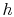,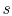, etc. are true state variables and that the 1st and 2nd laws of thermodynamics hold when the working medium is not an ideal gas (i.e. a two-phase medium). An elegant way to do this is to consider a Carnot cycle for a two-phase medium. To state the fact that all Carnot engines operated between two given temperatures have the same efficiency is one way of stating the 2nd law of thermodynamics. The working fluid need not be an ideal gas and may be a two-phase medium changing phases.

The idea is to run a Carnot engine between temperaturesand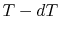for a two-phase medium and to let it undergo a change in phase. We can then derive an important relation known as the Clausius-Clapeyron equation, which gives the slope of the vapor pressure curve. We could then measure the vapor pressure curve for various substances and compare the measured slope to the Clausius-Clapeyron equation. This can then be viewed as an experimental proof of the general validity of the 1st and 2nd laws of thermodynamics!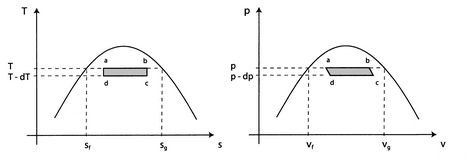Consider the infinitesimal Carnot cycleshown in Figure 8.8. Heat is absorbed between states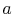and. To vaporize an arbitrary amount of mass,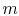, the amount of heat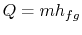(8..1)

must be supplied to the system. From the 1st and 2nd laws of thermodynamics the thermal efficiency for a Carnot cycle can be written as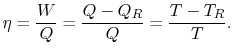Hence, for the infinitesimal cycle considered above,(8..2)

The work along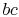andnearly cancel such that the net work is the difference between the work alongand, and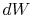can be viewed as the area enclosed by the rectangle: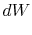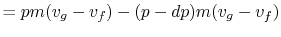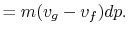(8..3)

Substituting Equations (8.1) and (8.3) into (8.2) one obtainsRearranging terms yields the Clausius-Clapeyron equation, which defines the slope of the vapor pressure curve: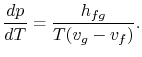(8..4)

The beauty is that we have found a general relation between experimentally measurable quantities from first principles (1st and 2nd laws of thermodynamics).

In order to plot the Clausius-Clapeyron relation and to compare it against experimentally measured vapor pressure curves, we need to integrate Equation (8.4). To do so, the heat of vaporization and the specific volumes must be known functions of temperature. This is an important problem in physical chemistry but we shall not pursue it further here except to mention that if

• variations in heat of vaporization can be neglected,
• the vapor phase is assumed to be an ideal gas, and
• the specific volume of the liquid is small compared to that of the vapor phase,the integration can be readily carried out8.1. Making these approximations, the Clausius-Clapeyron equation becomesCarrying out the integration, the resulting expression is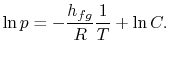Note that the vapor pressure curves are straight lines if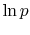is plotted versusand that the slope of the curves is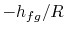, directly related to the heat of vaporization. Figures 8.9, 8.9, and 8.22 depict the vapor pressure curves for various substances. The fact that all known substances in the two-phase region fulfill the Clausius-Clapeyron equation provides the general validity of the 1st and 2nd laws of thermodynamics!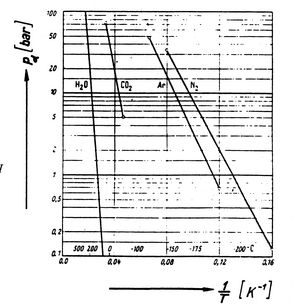UnifiedTP